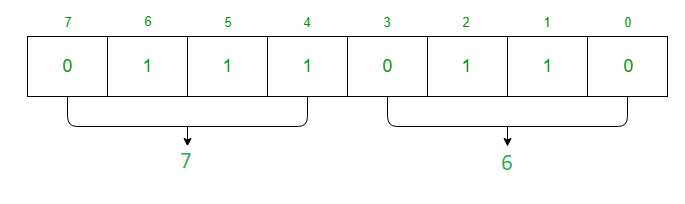Related Articles
Interface 8254 PIT with 8085 microprocessor
• Last Updated : 23 Aug, 2018

Prerequisite – 8254 Control Register and Operating modes
Problem – Write an assembly language program in 8085 microprocessor which generates 1 KHz square waveform by using counter 1 as a binary counter if clock frequency of 8254 is 2 MHz.

Assumption – Assume the port addresses are 80 H, 81 H, 82 H, 83 H for C0(Counter 0), C1(Counter 1), C2(Counter 2), CR(Control Register).

For the above problem, 8254 must work in Mode 3 which is the square wave generator.
Count for register is given as clock frequency / square wave frequency
count = 2 MHz / 1 KHz
= 2000
= (07D0) H
Now the data is 16 bit so value of RW1 = 1 and RW0 = 1 in Control Register. As we want to select C1 (Counter 1) the value of SC1 = 0 and SC0 = 1 in Control Register. Value of M2 = 0, M1 = 1 and M2 = 1 for Mode 3 in Control Register. For binary counter value of LSB in CR is 0.

Hence the Control Register(CR) is given by,Algorithm –

1. Move the data 76 in A
2. Display the contents of A to port 83
3. Move the data D0 in A
4. Display the contents of A to port 81
5. Move the data 07 in A
6. Display the contents of A to port 81
7. Stop

Program –

2000MVI A 76A <- 76
2002OUT 83CR <- A
2004MVI A D0A <- D02006OUT 81C1 <- A2008MVI A 07A <- 07200AOUT 81C1 <- A200CHLTStop

Explanation–

1. MVI A 76 is used to move the content of CR(Control Register) to register A.
2. OUT 83 is used to assign the value of A to port 83 which is Control Register.
3. MVI A D0 is used to move the move the lower byte of data of Counter 1 to register A.
4. OUT 81 is used to assign the value of A to port 81 which is Counter 1.
5. MVI A 07 is used to move the move the higher byte of data of Counter 1 to register A.
6. OUT 81 is used to assign the value of A to port 81 which is Counter 1.
7. HLT is used end the program.
My Personal Notes arrow_drop_up